# CBSE Class 9 Science Structure Of Atom Notes Set B

Download CBSE Class 9 Science Structure Of Atom Notes Set B in PDF format. All Revision notes for Class 9 Science have been designed as per the latest syllabus and updated chapters given in your textbook for Science in Standard 9. Our teachers have designed these concept notes for the benefit of Grade 9 students. You should use these chapter wise notes for revision on daily basis. These study notes can also be used for learning each chapter and its important and difficult topics or revision just before your exams to help you get better scores in upcoming examinations, You can also use Printable notes for Class 9 Science for faster revision of difficult topics and get higher rank. After reading these notes also refer to MCQ questions for Class 9 Science given our website

STRUCTURE OF ATOM

INTRODUCTION

(i) The idea of tiniest unit of matter (Anu and Parmanu) was propounded by maharishi Kanad in Vedic period in our country.

(ii) Democritus, a Greek philosopher also proposed that matter is made up of extremely small particles, the             “atom”. The name atom comes from Greek language.

(iii) John Dalton in 1808 published theory of atom assuming that atoms are the ultimate indivisible particles of matter.

(iv) Later the works f William Crookes (1878) , J.J. Thomson (1897) and Goldstein proved that atom of any element contains smaller particles which are either positively charged or negatively charged.

(v) Work of Rutherford and Neils Bohr confirmed that an atom consists of three subatomic particles, that are electrons, protons and neutrons.

(vi) It has been established that the central core of an atom consists of protons and neutrons and is commonly called nucleus. The electrons revolve around the nucleus.

(vii) The atom as a whole is electrically neutral as the number of protons in it, is equal to the number of electrons.

The smallest indivisible particle or unit of an element is called an atom, which can take part in a chemical reaction and may or may not exist independently.

An element is a pure substance which cannot be subdivided into two or more new substances by any means.

The word “ATOM” is given by “John Dalton”.

FUNDAMENTAL PARTICLES OF AN ATOM

(a) Electron :

Electron has a negative charge on it, its mass is 1/1837 times the mass of one atom of hydrogen. It is denoted by the symbol   -1 θ, where 0 denotes its mass and -1 denotes its charge. Electrons in the outer most shell are called valence electrons.

(b) Proton :

Proton has a unit positive charge, it is denoted by the symbol 1+1 p, where 1 denotes it atomic mass and +1 denotes its charge.

(c) Neutron :

Neutron has no electric charge on it. Its mass is almost equal to the mass of one atom of hydrogen. it is denoted by the symbol 10 n, where 1 denotes its atomic mass and 0 denotes its charge.

In the neutral atom the total number of protons in the nucleus is equal to the number of electrons revolving round the nucleus.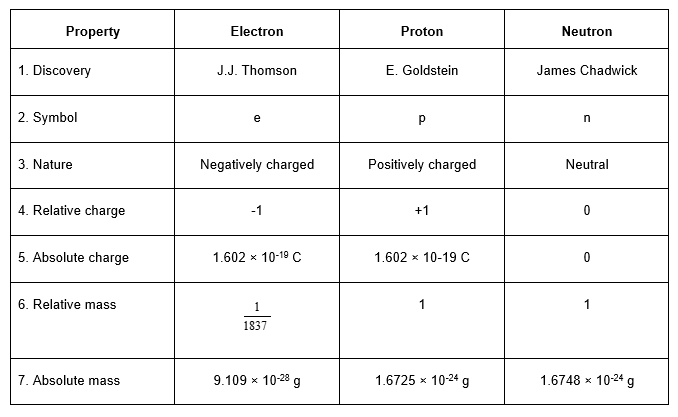THOMSONS MODEL OF AN ATOM

After the discovery of electrons and protons J.J. Thomson (1898) tried to explain the arrangement of electrons and protons within the tom. He proposed that an atom consists of a sphere of positive electricity in which electrons are embedded like plum in pudding or seeds evenly distributed in red spongy mass in watermelon. The radius of the sphere is of the other 10-8 cm which is equal to the size of the atom. Although Thmoson’s model could explain the electrical neutrality of an atom but this model could not satisfy experimental facts proposed by Rutherford and hence was discarded.“Proton is a sub - atomic particle having a unit positive charge (+1.602 × 10-19 kg) & mass (1.6725 × 10-27 kg) which is about 1837 times greater than the mass of an electron.”

RUTHERFORD’S MODEL OF AN ATOM

(a) Rutherford’s Alpha Scattering Experiment :

Ernest Rutherford and his coworkers performed numerous experiments in which  particles emitted from a radioactive element such as polonium were allowed to strike thin sheets of metals such as gold and platinum.

(i) A beam of -particles (He2+) we obtained by placing polonium in a lead box and letting the alpha particles come out of a pinhole in the lead box. This beam of -rays was directed against a thin gold foil (0.00004 cm). A circular screen coated with zinc sulphide was placed on the other side of the foil.

(ii) About 99% of the  particles passed undeflected through the gold foil and caused illumination of zinc sulphide screen.

(iii) Very few -particles underwent small and large deflections after passing through the gold foil.

(iv) A very few (about 1 in 20,000) were deflected backward on their path at an angle of 1800.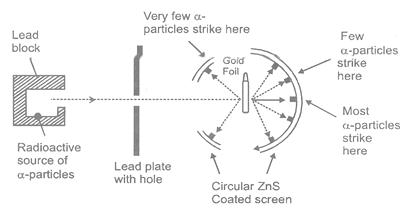Rutherford was able to explain these observations as follows :

(i) Since a large number of -particles pass through the atom undeflected, hence, there must be large empty space within the atom.

(ii) As some of the - particles got defleted, therefore, there must be something massive and positively charged present in the atom.

(iii) The number of -particles which got deflected is very small, therefore, the whole positive charge in the atom is conventratted in a very small space.

(iv) Some of the -particles retracted their path i.e. came almost straight back towards the sources as a result of their direct collisions with the heavy mass.

- particles are made up of two protons and two neutrons and are He nuclei.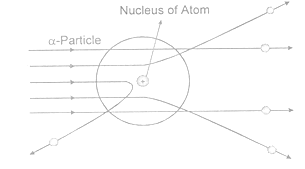The positively charged heavy mass which occupies only a small space as compared to the total space occupied by the atom is called nucleus.

(b) Rutherford’s Nuclear Model of Atom :

Rutherford proposed a new picture of the structure of the atom. Main feature of this model are as follows -

(i) The atom of an element consists of a small positively charged “Nucleus” which is situated at the centre of the atom and which carries almost the entire mss of the atom.

(ii) The electrons are distributed in the empty space of the atom around the nucleus in different concentric circular paths (orbits).

(iii) The number of electrons in the orbits is equal to the total number of protons in the nucleus.

(iv) Volume of nucleus is very small as compared to the volume of atom.

(v) Most of the space in the atom is empty.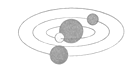The stability of such a system in which negatively charged electrons surround a positively charged nucleus was explained by proposing that the electrons revolve around the nucleus at very high speed in circular orbits. This arrangement is just like our solar system. The high sped of the moving electrons given them a centrifugal force acting away from the nucleus. The centrifugal force balance the electrostatic force of attraction acting between the nucleus and the electrons.

(c) Defects in Rutherford’s Model :

(i) Rutherford did not specify the number of electrons in each orbit.

(ii) According to electromagnetic theory, if a charged particle (electron) is accelerated around another charged particle (nucleus) then there would be continuous radiation of energy. This loss of energy would slow down the  speed of electron and eventually the electron would fall into the nucleus. But such a collapse does not occur. Rutherford’s model could not explain this theory.

BOHR’S MODEL OF AN ATOM

Rutherford’s model of the atom was unable to explain certain observations with regard to the atom i.e. stability of the atom and the occurrence of the atomic spectra. Neils Bohr accepted Rutherford’s idea that the positive charge and most of the mass of the atom is concentrated in its nucleus with the electrons present at some distance away.

According to Bohr’s theory -

(i) Electrons revolve around the nucleus in well defined orbits or shells, each shell having a definite amount of energy associated with the electrons in it. Therefore, these shells are also called energy levels.

(ii) The energy associated with the electrons in an orbit increases as the radius of the orbit increases. These shells are known as K, L, M, N etc. starting from the one closest to the nucleus.

(iii) An electron in a shell can more to a higher or lower energy shell by absorbing or releasing a fixed amount of energy.

(iv) The amount of energy absorbed or emitted is given by the difference of energies associated with the two energy levels.

Energy absorbed,        DE = E2 - E1 =  hν

Energy emitted,           DE = E2 - E1 = hν

Where h is Plank’s constant (h = 6.62 × 10-34 Js) and  is the frequency of the radiation.ATOMIC STRUCTURE

An atom consists of two parts :

(a) Nucleus :

Nucleus is situated in the centre of an atom. All the protons & neutrons are situated in the nucleus, therefore, the entire mass of an atom is almost concentrated in the nucleus. The overall charge of nucleus is positive due to the presence of positively charged protons (neutrons present have no charge). The protons & neutrons are collectively called nucleons. The radius of the nucleus of an atom is of the order of 10-15 m.

(b) Extra nuclear region :

In extra nuclear part or in the region outside the nucleus, electrons are present which revolve around the nucleus in orbits of fixed energies. These orbits are called energy levels. These energy levels are designated as K, L, M, N and so on.

The maximum number of electrons that can be accommodated in a shell is given by the formula 2n2 (where n = number of shell i.e. 1, 2, 3--)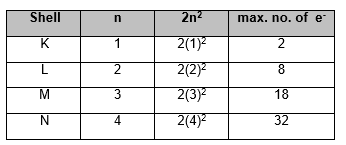Each energy level is further divided into subshells designated as s.p.d.f.

1st shell (K) contains 1 subshell (s)

2nd shell (L) contains 2 subshells (s,p)

3rd shell ( M) contains 3 subshells (s,p,d)

4th shell (N) contains 4 subshells (s,p,d,f).

ORBITALS

Like shells are divided into subshells, subshells further contain orbitals.

An orbital may be defined as a

“Region in the three - dimensional space around the nucleus where the probability of finding an electron is maximum. The maximum capacity of each orbital is that of two electrons.”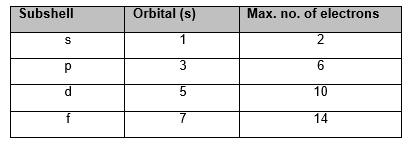The total number of nucleons is equal to the mass number (A) of the atom.

ELECTRONIC CONFIGUATION OF AN ATOM

(i) Each of the orbits can accommodate a fixed number of electrons. Maximum number of electrons in an orbit is equal to 2n2, where ‘n’ is the number of the orbit.

If n = 1 then 2n2 = 2

n = 2 then 2n2 = 8

n = 3 then 2n2 = 18

n = 4 then 2n2 = 32

(ii) In the outermost shell of any atom, the maximum possible number of electrons is 8, except in the first shell which can have at the most 2 electrons.

(iii) The arrangement of the electrons is different shells is known as the electronic configuration of the element. The pictorial representations of Bohr’s model o hydrogen, helium, carbon, sodium and calcium atoms having 1, 2, 6, 11 and 20 electrons respectively are shown in the figure where the centre of the circle represents the nucleus.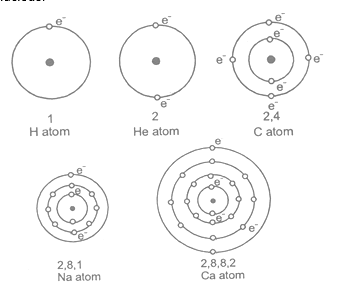If the outermost shell has 8 electrons it is said to be an octet. If the first shell has its full quota of 2 electrons, it is said to be duplet.

ELECTRONIC CONFIGURATION OF ELEMENTS UPTO ATOMIC NUMBER 30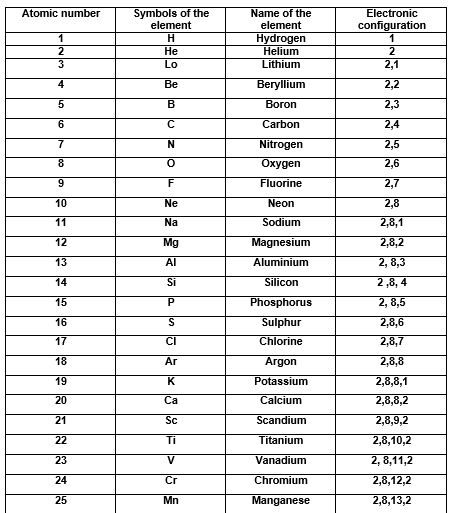VALENCY

Valency of an element is the combining capacity of the atoms of the element with atoms of the same or different elements. The combining capacity of the atoms of other elements was explained in terms of their tendency to attain a fully - filled outermost shell (stable octet or duplet).

The number of electrons gained, lost or contributed for sharing by an atom of the element gives us directly the combining capacity or valency of the element.

Valency of an element is determined by the number of valence electrons in an atom of the element.

The valency of an element        =          number of valence electrons

(when number of valence electrons are from 1 to 4)

The valency of an element        =          8 - number of valence electrons.

(when number of valence electrons are more than 4)

Na has 1 valence electron, thus, its valency is 1.

Cl has 7 valence electrons, thus, its valency is 8 - 7 = 1.

ATOMIC NUMBER (Z)

The number of protons is the nucleus of an atom of a given element is called the atomic number of that element.

or

Atomic number is the number of protons present in the atom of an element. It is denoted. by “Z”

Atomic number = Number of protons = Number of electrons ( in a neutral atom)

Atomic number = Number of protons    (in an ion)

e.g. 11Na

-Atomic number of sodium is 11

-Nucleus of sodium has 11 protons.

- Nucleus of sodium has 11 units of positive charge.

- There are 11 electrons, revolving round the nucleus of sodium.

The atomic number is represented on the LHS of the symbol of the element as subscript.

MASS NUMBER (A)

Mass number is the number of protons and neutrons present in the atom of an element. It is denoted by “A”. The mass number is represented either on the left hand side (LHS) or on the right hand side (RHS) of the symbol of the element as superscript.

Mass number = Number of protons + Number of neutrons.

e.g.

-Mass number of aluminum is 27.

-The total number of protons and neutrons is the nucleus of aluminum is 27.

-Number of protons is 13.

-Number of neutrons is = 27 - 13 = 14.

Each element has a unique atomic number which is its identity.

(a) Relation between Z, A and N

A = Z + N

Z = Number of Protons

N = Number of neutrons

A = Mass number

N = A - Z

ISOTOPES

(a) Atoms of same element having the same chemical properties, but differing in mass are known as isotopes.

The isotopes of an element have the same atomic number but different atomic masses. Isotopes have the same electrical charges means same number of protons. The difference in their masses is due to the presence of different number of neutrons.

e.g. (a) Isotopes of hydrogen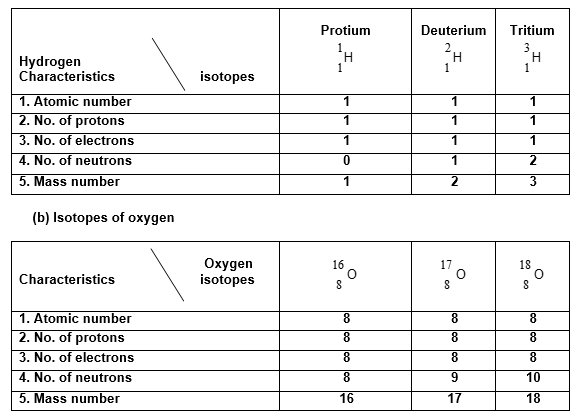All the isotopes of an element have identical chemical properties.

(b) Characteristics of Isotopes :

(i) The physical properties of the isotopes of an element are different number of neutrons in their nuclei. Hence mass, density and other physical properties of the isotopes of an element are different.

(ii) All the isotopes of an element contains the same number of electrons. So, they have the same electronic configuration with the same number of valence electrons. Since the chemical properties of an element are determined by the number of valence electrons in its atom, all the isotopes of an element have identical chemical properties.

(c) Reason for the fractional atomic masses of elements :

The atomic masses of many elements are in fraction and not whole number. The fractional atomic masses of elements are due to the existence of their isotopes having different masses.

e.g. :The atomic mass of chlorine is 35.5 u. Chlorine has two isotopeswith abundance of 75% and 25% respectively. Thus the average mass of a chlorine atom will be 75% of Cl - 35 and 25% of Cl-37, which is 35.5 u.

i.e., average atomic mass of chlorineThus, the average atomic mass of chlorine is 35.5 u.

Similarly, average atomic mass of copper is 63.5 u.

(d) Applications of Radioactive isotopes :

(i) In agriculture : Certain elements such as boron, cobalt, copper, manganese, zinc and molybdenum are necessary is very minute quantities for plant nutrition.

By radioactive isotopes we van identify the presence and requirements of these element in the nutrition of plants.

(ii) In industry : Coating on the arm of clock to seen in dark. To identify the cracks in metal casting.

(iii) In medicine : Thyroid, bone diseases, brain tumours and cancer and diagnosed, controlled or destroyed with the help of radioactive isotopes like,Na, iodine, phosphorus etc.

(iv) Determination of the mechanism of chemical reaction : by replacing an atom or molecule by its isotope.

(v) In carbon dating : Will and Libby (1960) developed the technique of radiocarbon dating to determine the age of plants, fossils and archeological samples.

Isotopes (Like Uranium - 238) are used in nuclear reactor to produce energy and power.

ISOBARS

the atoms of different elements with different atomic numbers, but same mass number are called isobars.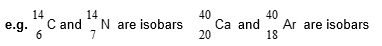ISOTONES

The isotones may be defined as the atoms of different elements containing same number of neutrons.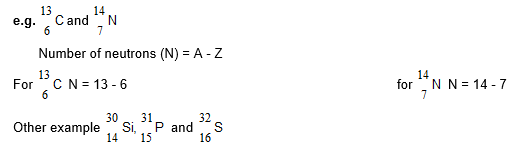ISOELECTRIC

Ion or atom or molecule which have the same number of electrons are called as isoelectronic species.

e.g.                              Cl-                    Ar                     K+                     Ca+2

No. of electrons         18                     18                     18                     18

Isobars contain different number of electrons, protons and neutrons

SOLVED EXAMPLES

1Calculate the number of electrons, protons and neutrons in the following species.

(i) Phosphorus atom      (ii) Phosphide ion (P3-)   (iii) Magnesium ion (Mg2+)

Mass number :             P = 31, Mg = 24

Atomic numbers :          P = 15, Mg = 12

Sol. (i) Phosphorus atom

Number of electrons = Atomic number = 15

Number of protons = Atomic number = 15

Number of neutrons = Mass number - Atomic number = 31 - 15 = 13.

(ii) Phosphide ion (P3-).

Phosphide ion (P3-) = Phosphorus atom + 3 electrons

P3-  P + 3e -

Thus, phosphide ion has same number of protons and neutrons as phosphorus atom but has three electrons more.

Number of electrons                  =          15 + 3 = 18

Number of protons                    =          15

Number of neutrons                   =          31 - 15 = 16

(iii) Magnesium ion (Mg2+)

Mg2+ ion is formed by the loss of two electrons by Mg atom. Therefore, it has two electrons less than the number of electrons is Mg atom.

Number of electrons                  =          12 - 2 = 10

Number of protons                    =          12

Number of neutrons                   =          (24 - 12_ = 12

2.The number of protons in the nucleus of an atom of mass number 97 is 41. What will be the number of neutrons in its isotope of mass number 99 ?

Sol.  The atomic number of isotopes is same. Therefore, the number of protons in both the atoms is same.

Mass number                           =          Number of protons + Number of neutrons

Number of neutrons              =          Mass number - Number of protons

=          99 - 41 = 58

3.Give number of protons and neutrons in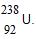Sol. From the given symbol it is clear that the atomic number of uranium is 92 and its mass number is 238.

Now, number of protons            =          Atomic number = 92

Number of neutrons                 =          Mass number - Atomic number

=          238 - 92 = 146

4.Calculate the atomic number of an element whose mass number is 31 and number of neutrons is 13. What is the symbol of the element ?

Sol. We know that, mass number = Number of protons + Number of neutrons

But number of protons is equal to the atomic number.

Mass number                     =          Atomic number + number of neutron

or    Atomic number                   =          Mass number - number of neutron = 31 - 16 = 15

The element with atomic number 15 is phosphorus which has symbol P.

5.If bromine atom is available in the form of, say two isotopes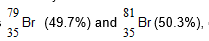, calculate the average atomic mass of bromine atom.

Sol.  % of Br (79) = 49.7 ; % of Br (81) = 50.3

Atomic mass of Br =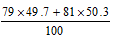=          80.0

Thus, atomic mass of bromine is 80.0

6.An isotope has atomic number 17 and mass number 37. What is the arrangement of electrons in the shells of this isotope ? State nuclear composition of this isotope.

Sol. Number of electrons      =          Atomic number   = 17

Number of protons        =             Atomic number        = 17

Number of neutrons     =          Mass number - Atomic number = 37 - 17 = 20

Electronic configuration of the isotope is         K          L          M

2          8          7

Nucleus of the isotope contains  17 protons  and 20 neutrons.

7.An element has 2 electrons is the M-shell. What is the electronic configuration of the element and what is its atomic number ?

Sol. The 2 electrons in M-shell indicates that the K and L - shell must be full. K - shell can accommodate a maximum of 2 electrons while L-shell can accommodate a maximum of 8 electrons. Thus, the electronic configuration of the element may be written as :

K          L          M

2           8          2

The total number of electrons is an atom of the element is 2 + 8 + 2 = 12

Therefore, atomic number of element is 12.

8.How will you find the valency of chlorine, sulphur and magnesium ?

Sol. (i) The atomic number of Cl is 17. Its electronic configuration is

K                L          M

2                8          7

Cl has 7 electrons in the valence shell. It needs one more electron to complete its octet. Hence, its valency is 1.

(ii) The atomic number of S is 16. Its electronic configuration is

K                L          M

2                8          6

S has 6 electrons is the valence shell. It requires two more electrons to complete its octet. Hence, its valency is 2.

(iii) The atomic number of Mg is 12. Its electronic configuration is

K                L          M

2                8          2

Mg has only 2 electrons in the valence shell. By losing these 2 electrons it can attain octet of electrons in its outer most shell. Hence, it valency is 2.

## Tags:

Click for more Science Study Material
 CBSE Class 9 Science Atoms And Molecules Notes Set A CBSE Class 9 Science Atoms And Molecules Notes Set B CBSE Class 9 Science Categories Of Practical Skills Notes CBSE Class 9 Science Diversity In Living Organisms Notes Set A CBSE Class 9 Science Diversity In Living Organisms Notes Set B CBSE Class 9 Science Force And Laws Of Motion Notes Set A CBSE Class 9 Science Force And Laws Of Motion Notes Set B CBSE Class 9 Science Fundamental Unit Of Life Notes CBSE Class 9 Science Gravitation And Fluid Notes Set A CBSE Class 9 Science Gravitation And Fluid Notes Set B CBSE Class 9 Science Gravitation And Fluid Notes Set C CBSE Class 9 Science Improvement In Food Resources Notes Set A CBSE Class 9 Science Improvement In Food Resources Notes Set B CBSE Class 9 Science Is Matter Around Us Pure Notes Set A CBSE Class 9 Science Is Matter Around Us Pure Notes Set B CBSE Class 9 Science List Of Experiments CBSE Class 9 Science Matter In Our Surroundings Notes Set A CBSE Class 9 Science Matter In Our Surroundings Notes Set B CBSE Class 9 Science Motion Notes CBSE Class 9 Science Motion Notes Set A CBSE Class 9 Science Natural Resources Notes Set A CBSE Class 9 Science Natural Resources Notes Set B CBSE Class 9 Science Physical Science Notes CBSE Class 9 Science Revision Notes CBSE Class 9 Science Sound Notes CBSE Class 9 Science Structure Of Atom Notes Set A CBSE Class 9 Science Structure Of Atom Notes Set B CBSE Class 9 Science The Fundamental Unit Of Life Notes CBSE Class 9 Science Tissue Notes Set A CBSE Class 9 Science Tissue Notes Set B CBSE Class 9 Science Wave Motion And Sound Notes CBSE Class 9 Science Why Do We Fall Ill Notes Set A CBSE Class 9 Science Why Do We Fall Ill Notes Set B CBSE Class 9 Science Work Energy And Power Notes Set A CBSE Class 9 Science Work Energy And Power Notes Set B

## Latest NCERT & CBSE News

Read the latest news and announcements from NCERT and CBSE below. Important updates relating to your studies which will help you to keep yourself updated with latest happenings in school level education. Keep yourself updated with all latest news and also read articles from teachers which will help you to improve your studies, increase motivation level and promote faster learning

### CBSE Class 12 Evaluation Criteria 2021

SUMMARY OF THE POLICY FOR TABULATION OF MARKS FOR CLASS XII BOARD EXAMINATIONS 2021   1(a) Due to cancellation of the Board examinations, the assessment of theory portion of 80/70/60/50/30 marks will be done by the school based on the following:     Class...

### CBSE Board Examination Scheme of Assessment 2021 2022

A. Academic session to be divided into 2 Terms with approximately 50% syllabus in each term: The syllabus for the Academic session 2021-22 will be divided into 2 terms by following a systematic approach by looking into the interconnectivity of concepts and topics by...

### FAQs on Policy for Tabulation of Marks for Class 10 Board Exams

ON POLICY Question.1 How CBSE will declare the result of Class-X? Answer. Results of Class X Board will be declared on the basis of an objective criterion developed by the Board vide Notification no.CBSE/CE/2021 dated 01.05.2021. Question.2. If any candidate is not...